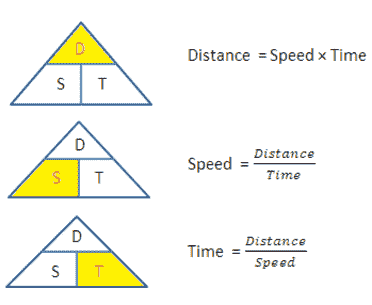# Calculate Average Speed using Distance and Time

The calculator which calculates the average speed based for entered values of time and distance.
 Distance: Miles Metres KM Yards Hours m/s Min: sec Sec: sec Average Speed: